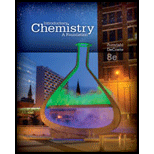# Some properties of potassium metal are summarized in the following table: rmal melting point :math&gt; 63 . 5 ° C rmal boiling point :math&gt; 765 . 7 ° C lar heat of fusion :math&gt; 2 . 334 kJ / mol lar heat of vaporization :math&gt; 79 . 87 kJ / mol ecific heat of the solid :math&gt; 0. 75 J / g ° C Calculate the quantity of heat required to heat 5 .00 g of potassium from 25 . 3 ° C to 45 . 2 ° C . Calculate the quantity of heat required to melt 1 . 35 moles of potassium at its normal melting point. Calculate the quantity of heat required to vaporize 2 . 25 g of potassium at its normal boiling point.### Introductory Chemistry: A Foundati...

8th Edition
Steven S. Zumdahl + 1 other
Publisher: Cengage Learning
ISBN: 9781285199030

#### Solutions

Chapter
Section### Introductory Chemistry: A Foundati...

8th Edition
Steven S. Zumdahl + 1 other
Publisher: Cengage Learning
ISBN: 9781285199030
Chapter 14, Problem 67AP
Textbook Problem
1 views

## Some properties of potassium metal are summarized in the following table:rmal melting point :math> 63 . 5  ° C rmal boiling point :math> 765 . 7  ° C lar heat of fusion :math> 2 . 334 kJ / mol lar heat of vaporization :math> 79 . 87 kJ / mol ecific heat of the solid :math> 0. 75 J / g  ° C Calculate the quantity of heat required to heat 5 .00  g of potassium from 25 . 3  ° C to 45 . 2  ° C . Calculate the quantity of heat required to melt 1 . 35 moles of potassium at its normal melting point. Calculate the quantity of heat required to vaporize 2 . 25 g of potassium at its normal boiling point.

Interpretation Introduction

(a)

Interpretation:

Quantity of heat required to heat 5.0g of potassium from 25.30C to 45.20C must be calculated.

Concept Introduction:

To increase the temperature of a solid we need to supply heat energy. Specific heat plays crucial role in increase of temperature of a substance.

### Explanation of Solution

The heat energy (H) required to increase temperature of a substance depends on mass of the substance (m), specific heat of the substance(s) and temperature rise (t). Thus we can write

H=m×s×t

Putting all the values we get

H=5g×0

Interpretation Introduction

(b)

Interpretation:

How much heat energy will be required to melt 1.35 mol of potassium at its normal melting point must be calculated?

Concept Introduction:

Melting point is the temperature at which a solid starts melting to liquid and until all the solid is not melted temperature remains same.

Interpretation Introduction

(c)

Interpretation:

How much heat energy will be required to vaporize 2.25g of potassium at its normal boiling point must be calculated?

Concept Introduction:

Boiling point is the temperature at which a liquid starts boiling to vapor and until all the liquid is not boiled off temperature remains same.

### Still sussing out bartleby?

Check out a sample textbook solution.

See a sample solution

#### The Solution to Your Study Problems

Bartleby provides explanations to thousands of textbook problems written by our experts, many with advanced degrees!

Get Started

Find more solutions based on key concepts
Vitamin C deficiency symptoms include: red spots. loose teeth. anemia. all of the above.

Nutrition: Concepts and Controversies - Standalone book (MindTap Course List)

Which amino acids have side-chain groups that can form salt bridges?

Chemistry for Today: General, Organic, and Biochemistry

How many autosomes are present in a body cell of a human being? In a gamete?

Human Heredity: Principles and Issues (MindTap Course List)

Are asteroid collisions the only causes of mass extinction events?

Oceanography: An Invitation To Marine Science, Loose-leaf Versin

Two inductors having inductances L1 and L2 are connected in parallel as shown in Figure P31.53a. The mutual ind...

Physics for Scientists and Engineers, Technology Update (No access codes included)